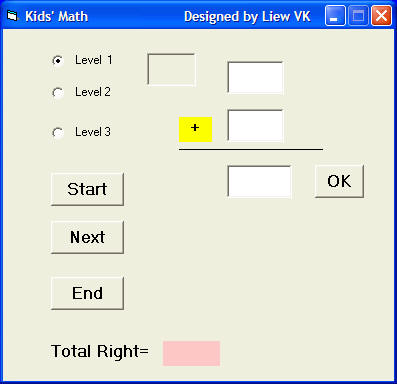# Kid's Math

This is a simple arithmetic educational game for children. The child who attempts the test can choose three different levels and perform three different arithmetic calculations. The performance can be evaluated by three measurements namely the total of questions attempted, the total of answers that are correct and the total score which is the percentage of right answers.

To generate the questions, we use the syntax Int(Rnd * n), where the Rnd function returns a  random value between 0 and 1, and Int is the function that converts a number into an integer by truncating its decimal decimal part and the resulting integer is the largest integer that is smaller than the number. For examples, let's say Rnd returns a value of 0.23245, then Rnd*10=2.3245, therefore Int(Rnd*10)=Int(2.3245)=2.

### The Interface### The Code

Dim num1 As Integer
Dim num2 As Integer
Dim intNumber As Integer

Private Sub score()
intNumber = intNumber + 1
End Sub

Private Sub begin()
intNumber = 0
Image1.Visible = False
Image2.Visible = False
Label6.Visible = False
Label5.Visible = False
Text3.Text = ""
If Option1.Value = "true" Then
n = 10
ElseIf Option2.Value = "true" Then
n = 100
ElseIf Option3.Value = "true" Then
n = 1000
End If

num1 = Int(Rnd * n)
num2 = Int(Rnd * n)
Text1.Text = num1
Text2.Text = num2
Text3.SetFocus

End Sub

Image1.Visible = False
Image2.Visible = False
Label6.Visible = False
Label5.Visible = False
End Sub

Private Sub Start_Click()
begin
End Sub

Private Sub text3_keyDown(keyCode As Integer, shift As Integer)
If keyCode = vbKeyNext Or keyCode = vbKeyDown Then
begin
End If
End Sub

Private Sub text3_keypress(keyAscii As Integer)
If (keyAscii = 13) And Val(Text3.Text) = Val(Text1.Text) + Val(Text2.Text) Then

Image1.Visible = True
Image2.Visible = False
Label5.Visible = True
Label6.Visible = False
score
total.Caption = Str\$(intNumber)

ElseIf (keyAscii = 13) And Val(Text3.Text) <> Val(Text1.Text) + Val(Text2.Text) Then
Image1.Visible = False
Image2.Visible = True
Label5.Visible = False
Label6.Visible = True
Text3.Text = ""
End If

End Sub

Private Sub Next_Click()
Image1.Visible = False
Image2.Visible = False
Label6.Visible = False
Label5.Visible = False
If Option1.Value = "true" Then
n = 10

ElseIf Option2.Value = "true" Then
n = 100
ElseIf Option3.Value = "true" Then
n = 1000
End If
Text1.Text = ""
Text2.Text = ""
Text3.Text = ""
num1 = Int(Rnd * n)
num2 = Int(Rnd * n)
Text1.Text = num1
Text2.Text = num2
Text3.SetFocus
End Sub

Private Sub OK_Click()
If Val(Text3.Text) = Val(Text1.Text) + Val(Text2.Text) Then
Image1.Visible = True
Image2.Visible = False
Label5.Visible = True
Label6.Visible = False
score
total.Caption = Str\$(intNumber)

Else
Image1.Visible = False
Image2.Visible = True
Label5.Visible = False
Label6.Visible = True
Text3.Text = ""
End If
End Sub

Private Sub Option1_Click()
n = 10
End Sub

Private Sub Option2_Click()
n = 100
End Sub

Private Sub Option3_Click()
n = 1000
End Sub